Скачать презентацию CS 395 T JFK Protocol in Applied Pi

bd57c84eb1e30abc19004b503528f67b.ppt

• Количество слайдов: 26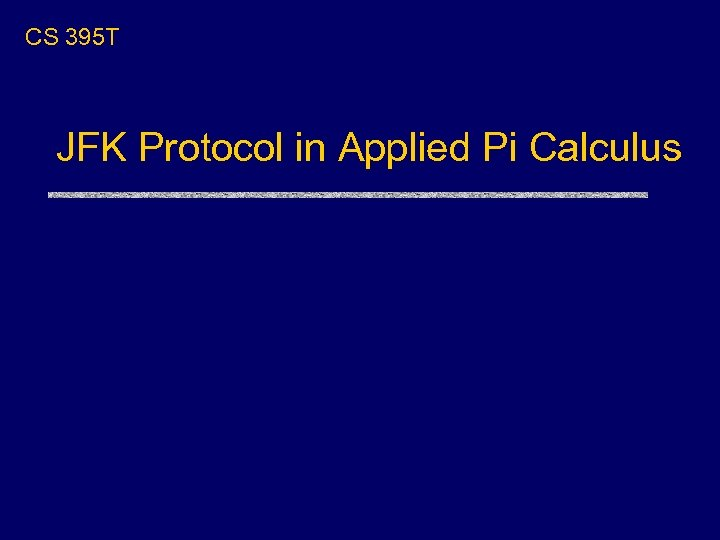CS 395 T JFK Protocol in Applied Pi Calculus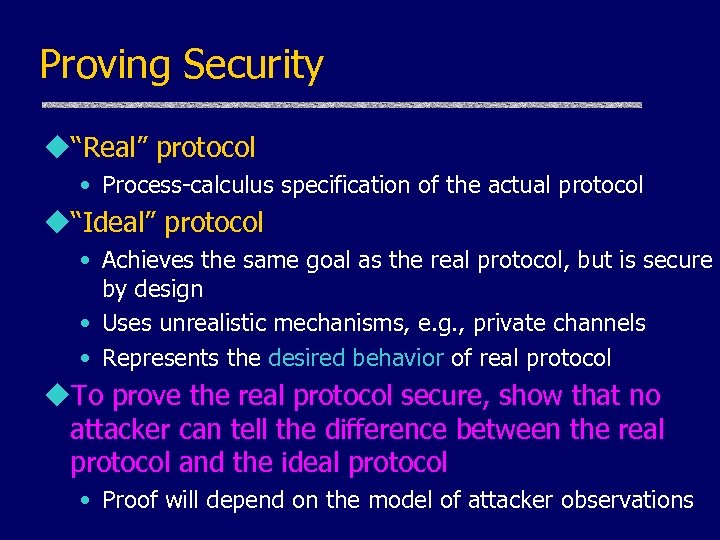Proving Security u“Real” protocol • Process-calculus specification of the actual protocol u“Ideal” protocol • Achieves the same goal as the real protocol, but is secure by design • Uses unrealistic mechanisms, e. g. , private channels • Represents the desired behavior of real protocol u. To prove the real protocol secure, show that no attacker can tell the difference between the real protocol and the ideal protocol • Proof will depend on the model of attacker observations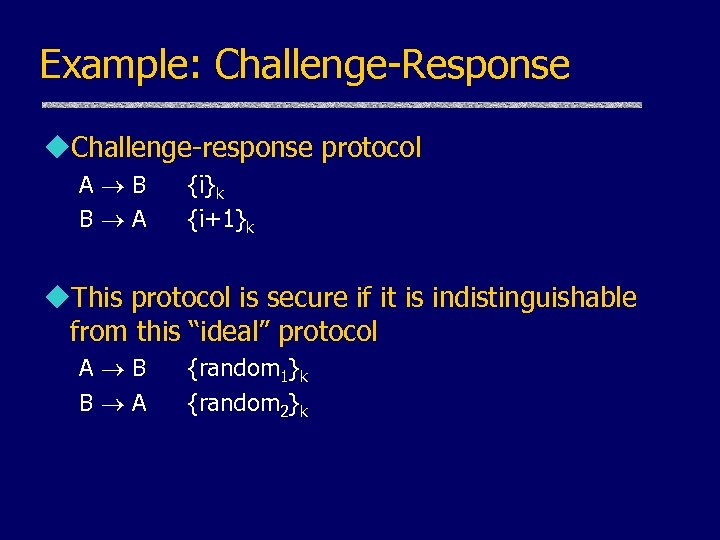Example: Challenge-Response u. Challenge-response protocol A B B A {i}k {i+1}k u. This protocol is secure if it is indistinguishable from this “ideal” protocol A B B A {random 1}k {random 2}k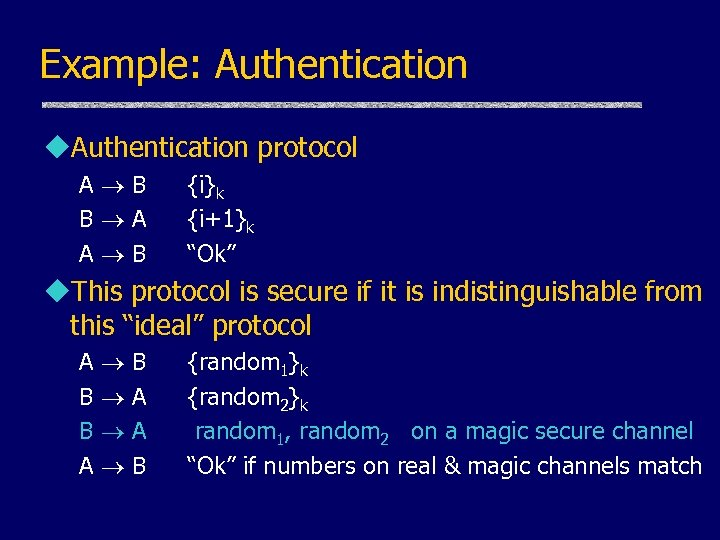Example: Authentication u. Authentication protocol A B B A A B {i}k {i+1}k “Ok” u. This protocol is secure if it is indistinguishable from this “ideal” protocol A B B A A B {random 1}k {random 2}k random 1, random 2 on a magic secure channel “Ok” if numbers on real & magic channels match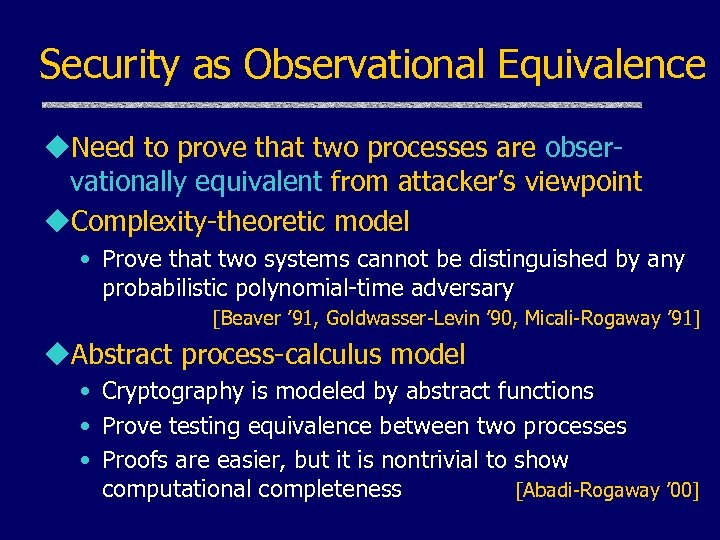Security as Observational Equivalence u. Need to prove that two processes are observationally equivalent from attacker’s viewpoint u. Complexity-theoretic model • Prove that two systems cannot be distinguished by any probabilistic polynomial-time adversary [Beaver ’ 91, Goldwasser-Levin ’ 90, Micali-Rogaway ’ 91] u. Abstract process-calculus model • Cryptography is modeled by abstract functions • Prove testing equivalence between two processes • Proofs are easier, but it is nontrivial to show computational completeness [Abadi-Rogaway ’ 00]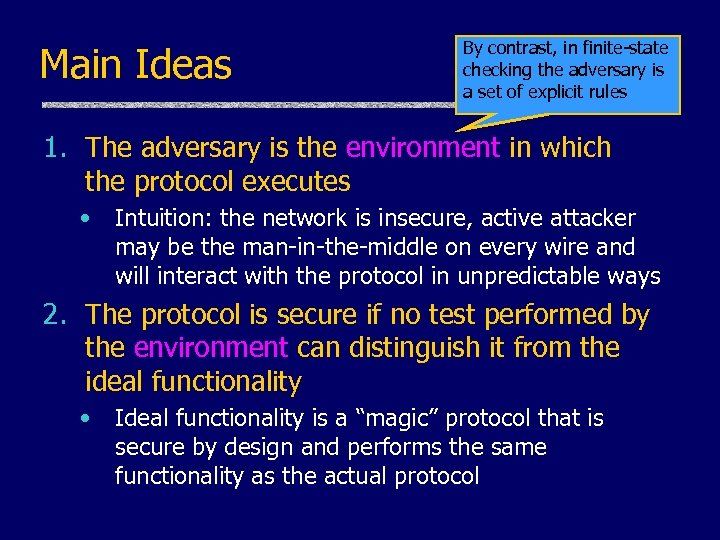Main Ideas By contrast, in finite-state checking the adversary is a set of explicit rules 1. The adversary is the environment in which the protocol executes • Intuition: the network is insecure, active attacker may be the man-in-the-middle on every wire and will interact with the protocol in unpredictable ways 2. The protocol is secure if no test performed by the environment can distinguish it from the ideal functionality • Ideal functionality is a “magic” protocol that is secure by design and performs the same functionality as the actual protocol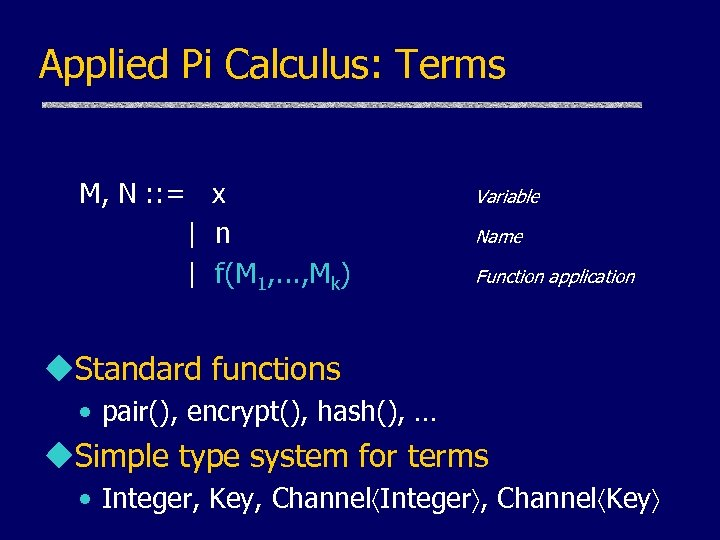Applied Pi Calculus: Terms M, N : : = x | n | f(M 1, . . . , Mk) Variable Name Function application u. Standard functions • pair(), encrypt(), hash(), … u. Simple type system for terms • Integer, Key, Channel Integer , Channel Key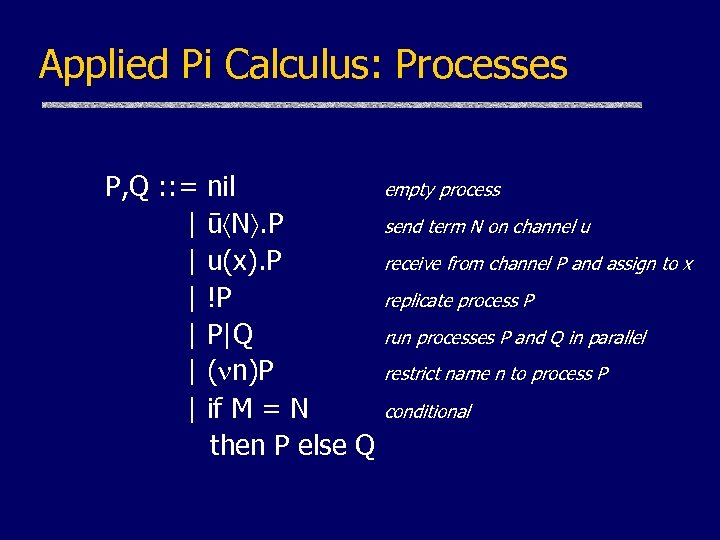Applied Pi Calculus: Processes P, Q : : = | | | nil ū N. P u(x). P !P P|Q ( n)P if M = N then P else Q empty process send term N on channel u receive from channel P and assign to x replicate process P run processes P and Q in parallel restrict name n to process P conditional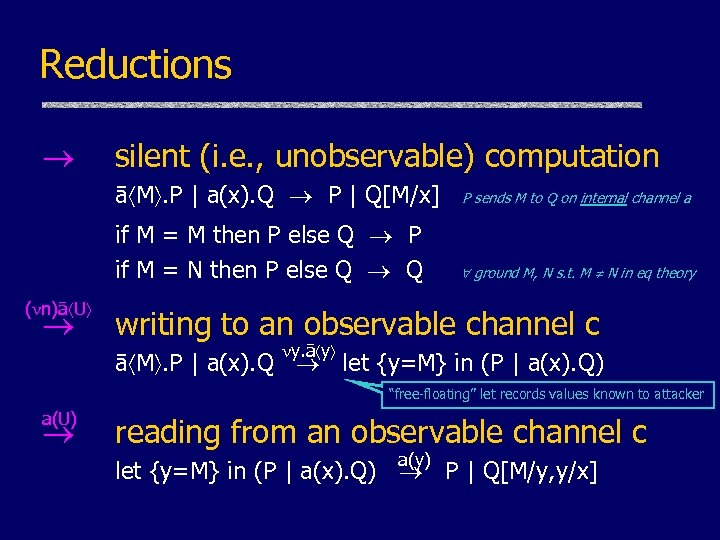Reductions silent (i. e. , unobservable) computation ā M. P | a(x). Q P | Q[M/x] if M = M then P else Q P if M = N then P else Q Q ( n)ā U P sends M to Q on internal channel a ground M, N s. t. M N in eq theory writing to an observable channel c y. ā y ā M. P | a(x). Q let {y=M} in (P | a(x). Q) “free-floating” let records values known to attacker a(U) reading from an observable channel c a(y) let {y=M} in (P | a(x). Q) P | Q[M/y, y/x]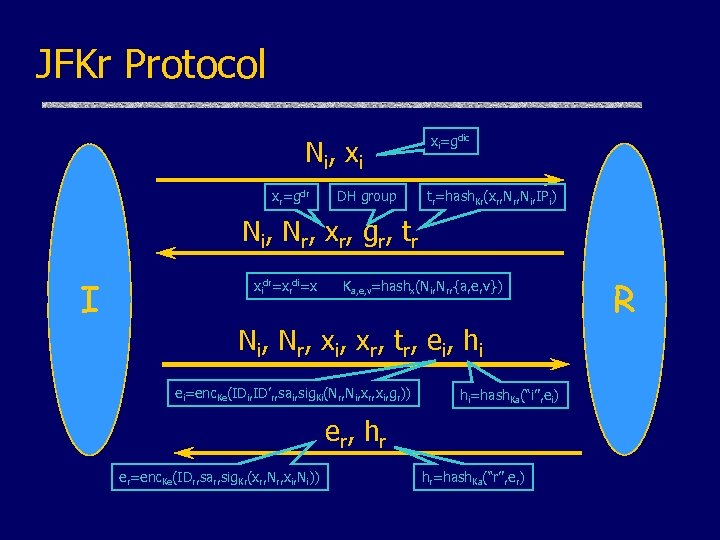JFKr Protocol N i, x i xr=gdr DH group xi=gdic tr=hash. Kr(xr, Ni, IPi) N i , N r, x r, g r, t r I xidr=xrdi=x Ka, e, v=hashx(Ni, Nr, {a, e, v}) N i , N r, x i , x r, t r, e i , h i ei=enc. Ke(IDi, ID’r, sai, sig. Ki(Nr, Ni, xr, xi, gr)) hi=hash. Ka(“i”, ei) e r, h r er=enc. Ke(IDr, sar, sig. Kr(xr, Nr, xi, Ni)) hr=hash. Ka(“r”, er) R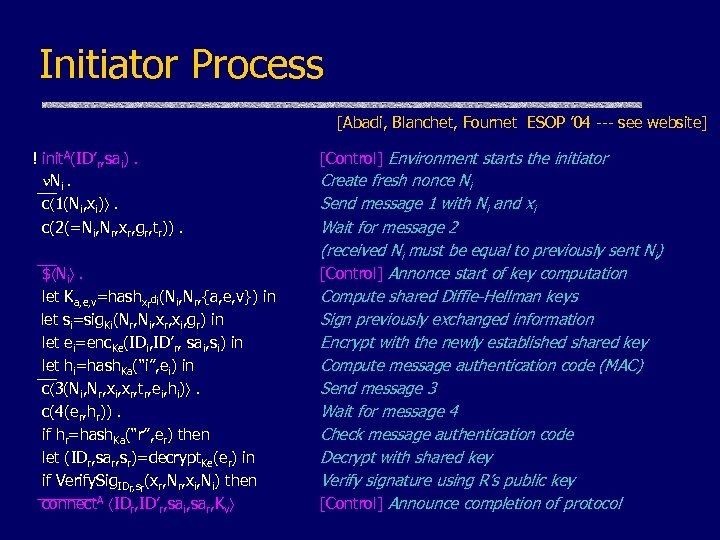Initiator Process [Abadi, Blanchet, Fournet ESOP ’ 04 --- see website] ! init. A(ID’r, sai). N __ i. c 1(Ni, xi) . c(2(=Ni, Nr, xr, gr, tr)). __ \$ Ni . let Ka, e, v=hashxrdi(Ni, Nr, {a, e, v}) in let si=sig. Ki(Nr, Ni, xr, xi, gr) in let ei=enc. Ke(IDi, ID’r, sai, si) in let __ hi=hash. Ka(“i”, ei) in c 3(Ni, Nr, xi, xr, tr, ei, hi) . c(4(er, hr)). if hr=hash. Ka(“r”, er) then let (IDr, sar, sr)=decrypt. Ke(er) in if Verify. Sig (x , N , x , N ) then ______ A IDr, sr r r i i connect IDr, ID’r, sai, sar, Kv [Control] Environment starts the initiator Create fresh nonce Ni Send message 1 with Ni and xi Wait for message 2 (received Ni must be equal to previously sent Ni) [Control] Annonce start of key computation Compute shared Diffie-Hellman keys Sign previously exchanged information Encrypt with the newly established shared key Compute message authentication code (MAC) Send message 3 Wait for message 4 Check message authentication code Decrypt with shared key Verify signature using R’s public key [Control] Announce completion of protocol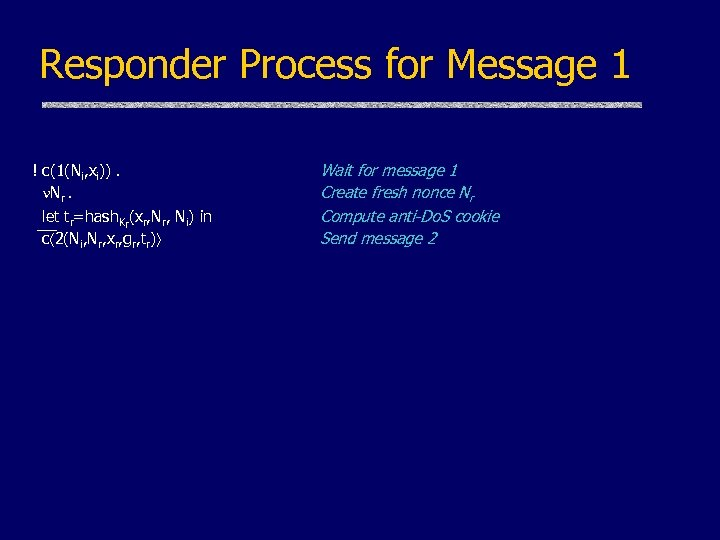Responder Process for Message 1 ! c(1(Ni, xi)). Nr. let __ tr=hash. Kr(xr, Ni) in c 2(Ni, Nr, xr, gr, tr) Wait for message 1 Create fresh nonce Nr Compute anti-Do. S cookie Send message 2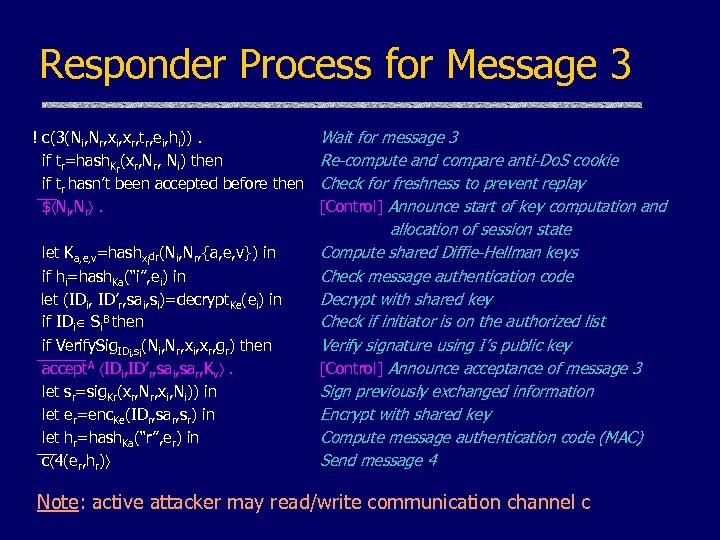Responder Process for Message 3 ! c(3(Ni, Nr, xi, xr, tr, ei, hi)). Wait for message 3 if tr=hash. Kr(xr, Ni) then Re-compute and compare anti-Do. S cookie if __tr hasn’t been accepted before then Check for freshness to prevent replay \$ Ni, Nr . [Control] Announce start of key computation and let Ka, e, v=hashxidr(Ni, Nr, {a, e, v}) in if hi=hash. Ka(“i”, ei) in let (IDi, ID’r, sai, si)=decrypt. Ke(ei) in if IDi Si. B then if Verify. Sig. IDi, si(Ni, Nr, xi, xr, gr) then _____ accept. A IDi, ID’r, sai, sar, Kv . let sr=sig. Kr(xr, Nr, xi, Ni)) in let er=enc. Ke(IDr, sar, sr) in let h =hash. Ka(“r”, er) in __ r c 4(er, hr) allocation of session state Compute shared Diffie-Hellman keys Check message authentication code Decrypt with shared key Check if initiator is on the authorized list Verify signature using I’s public key [Control] Announce acceptance of message 3 Sign previously exchanged information Encrypt with shared key Compute message authentication code (MAC) Send message 4 Note: active attacker may read/write communication channel c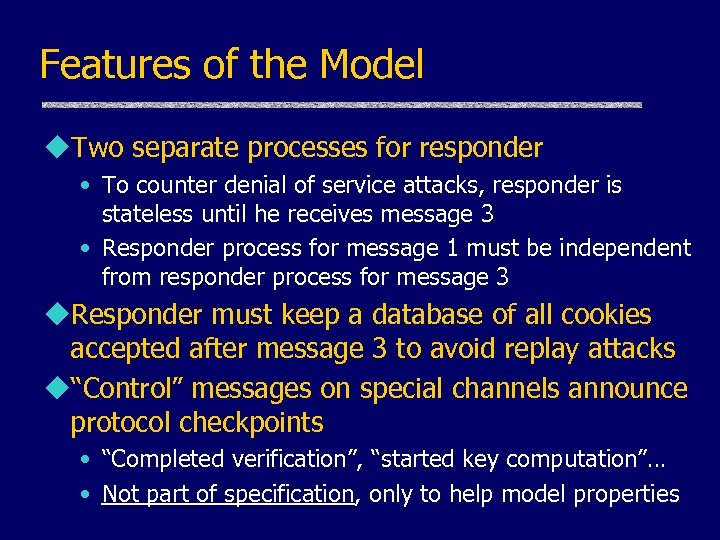Features of the Model u. Two separate processes for responder • To counter denial of service attacks, responder is stateless until he receives message 3 • Responder process for message 1 must be independent from responder process for message 3 u. Responder must keep a database of all cookies accepted after message 3 to avoid replay attacks u“Control” messages on special channels announce protocol checkpoints • “Completed verification”, “started key computation”… • Not part of specification, only to help model properties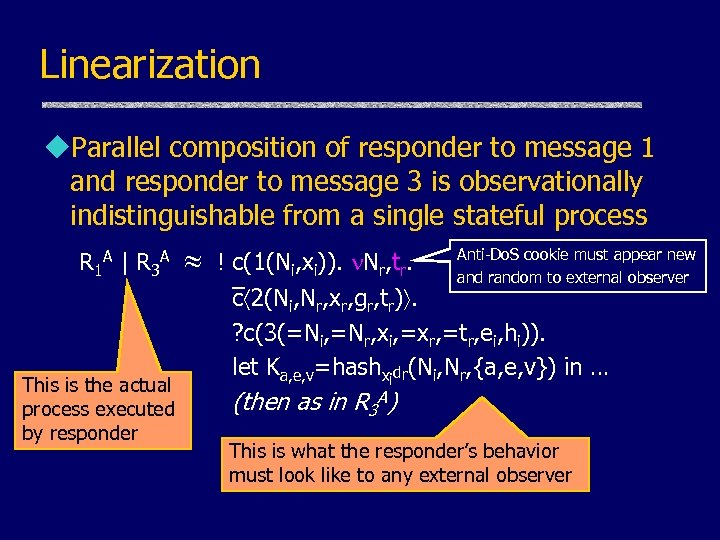Linearization u. Parallel composition of responder to message 1 and responder to message 3 is observationally indistinguishable from a single stateful process R 1 A | R 3 A This is the actual process executed by responder Anti-Do. S cookie must appear new ! c(1(Ni, xi)). Nr, tr. and random to external observer _ c 2(Ni, Nr, xr, gr, tr). ? c(3(=Ni, =Nr, xi, =xr, =tr, ei, hi)). let Ka, e, v=hashxidr(Ni, Nr, {a, e, v}) in … (then as in R 3 A) This is what the responder’s behavior must look like to any external observer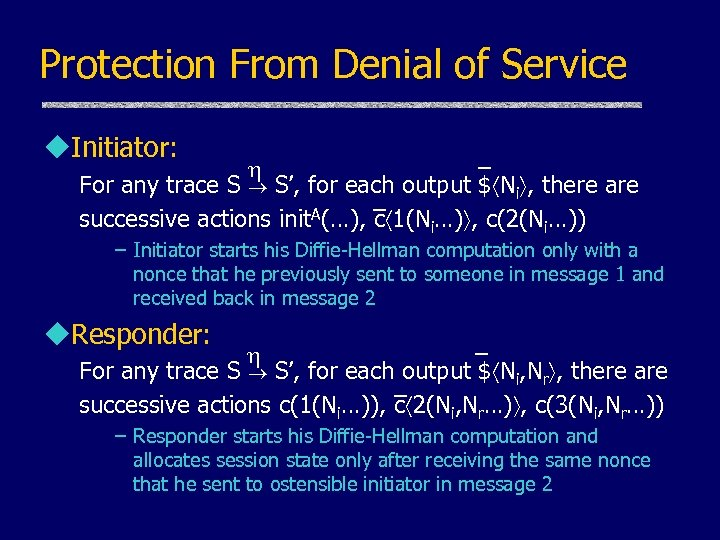Protection From Denial of Service u. Initiator: _ For any trace S S’, for each output \$ Ni , there are _ A(…), c 1(N …) , c(2(N …)) successive actions init i i – Initiator starts his Diffie-Hellman computation only with a nonce that he previously sent to someone in message 1 and received back in message 2 u. Responder: _ For any trace S S’, for each output \$ Ni, Nr , there are _ successive actions c(1(Ni…)), c 2(Ni, Nr…) , c(3(Ni, Nr…)) – Responder starts his Diffie-Hellman computation and allocates session state only after receiving the same nonce that he sent to ostensible initiator in message 2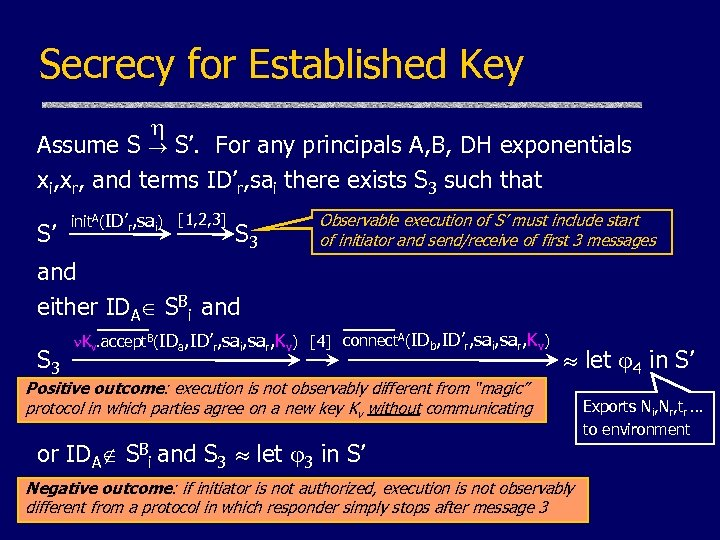Secrecy for Established Key Assume S S’. For any principals A, B, DH exponentials xi, xr, and terms ID’r, sai there exists S 3 such that S’ init. A(ID’r, sai) [1, 2, 3] S 3 and either IDA SBi and ____ S 3 Observable execution of S’ must include start of initiator and send/receive of first 3 messages ____ Kv. accept. B(IDa, ID’r, sai, sar, Kv)  connect. A(IDb, ID’r, sai, sar, Kv) let 4 in S’ Positive outcome: execution is not observably different from “magic” protocol in which parties agree on a new key Kv without communicating or IDA SBi and S 3 let 3 in S’ Negative outcome: if initiator is not authorized, execution is not observably different from a protocol in which responder simply stops after message 3 Exports Ni, Nr, tr … to environment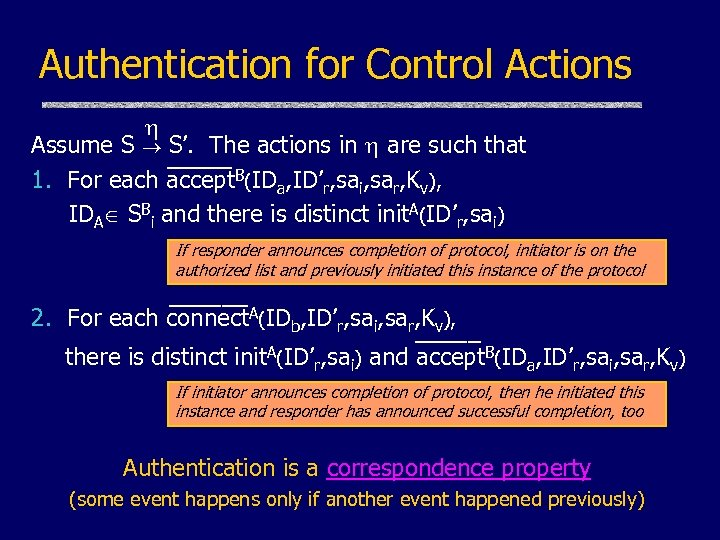Authentication for Control Actions Assume S S’. The actions in are such that _____ 1. For each accept. B(IDa, ID’r, sai, sar, Kv), IDA SBi and there is distinct init. A(ID’r, sai) If responder announces completion of protocol, initiator is on the authorized list and previously initiated this instance of the protocol ______ 2. For each connect. A(IDb, ID’r, sai, sar, Kv), _____ there is distinct init. A(ID’r, sai) and accept. B(IDa, ID’r, sai, sar, Kv) If initiator announces completion of protocol, then he initiated this instance and responder has announced successful completion, too Authentication is a correspondence property (some event happens only if another event happened previously)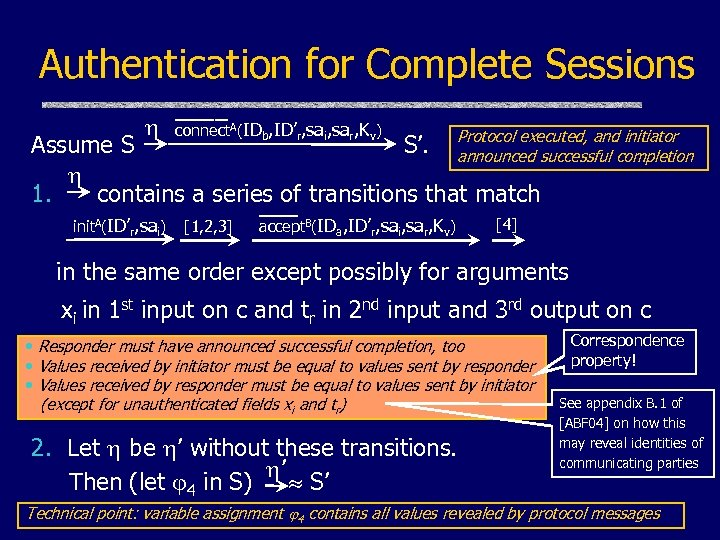Authentication for Complete Sessions ____ connect. A(IDb, ID’r, sai, sar, Kv) initiator Assume S S’. Protocol executed, andcompletion announced successful 1. contains a series of transitions that match ___ init. A(ID’r, sai) [1, 2, 3] accept. B(IDa, ID’r, sai, sar, Kv)  in the same order except possibly for arguments xi in 1 st input on c and tr in 2 nd input and 3 rd output on c • Responder must have announced successful completion, too • Values received by initiator must be equal to values sent by responder • Values received by responder must be equal to values sent by initiator (except for unauthenticated fields xi and tr) 2. Let be ’ without these transitions. ’ Then (let 4 in S) S’ Correspondence property! See appendix B. 1 of [ABF 04] on how this may reveal identities of communicating parties Technical point: variable assignment 4 contains all values revealed by protocol messages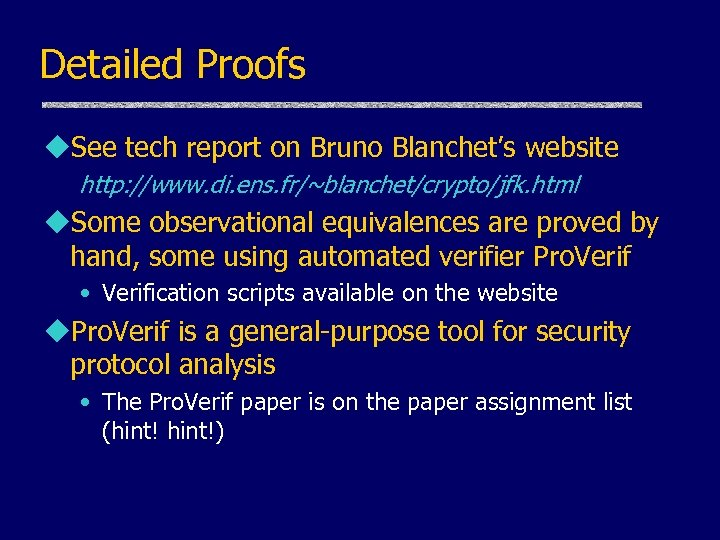Detailed Proofs u. See tech report on Bruno Blanchet’s website http: //www. di. ens. fr/~blanchet/crypto/jfk. html u. Some observational equivalences are proved by hand, some using automated verifier Pro. Verif • Verification scripts available on the website u. Pro. Verif is a general-purpose tool for security protocol analysis • The Pro. Verif paper is on the paper assignment list (hint!)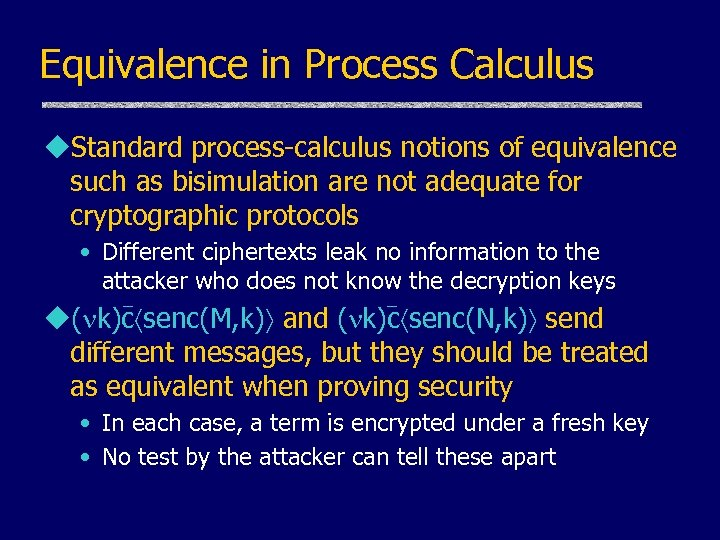Equivalence in Process Calculus u. Standard process-calculus notions of equivalence such as bisimulation are not adequate for cryptographic protocols • Different ciphertexts leak no information to the attacker who does not know the decryption keys - u( k)c senc(M, k) and ( k)c senc(N, k) send different messages, but they should be treated as equivalent when proving security • In each case, a term is encrypted under a fresh key • No test by the attacker can tell these apart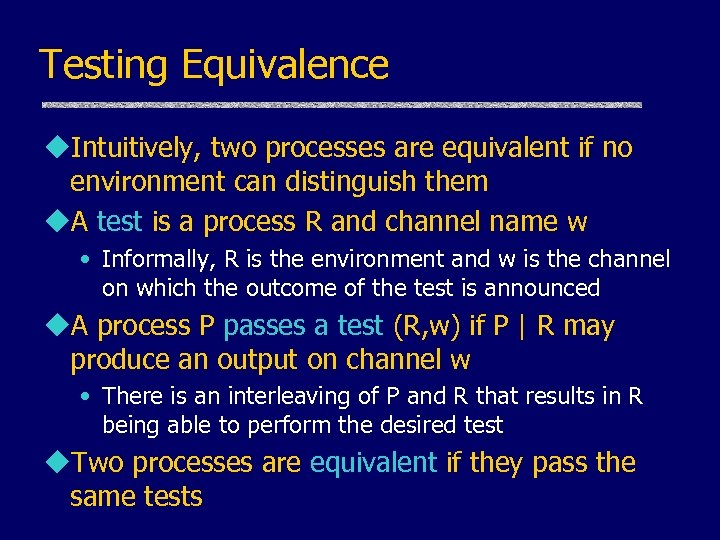Testing Equivalence u. Intuitively, two processes are equivalent if no environment can distinguish them u. A test is a process R and channel name w • Informally, R is the environment and w is the channel on which the outcome of the test is announced u. A process P passes a test (R, w) if P | R may produce an output on channel w • There is an interleaving of P and R that results in R being able to perform the desired test u. Two processes are equivalent if they pass the same tests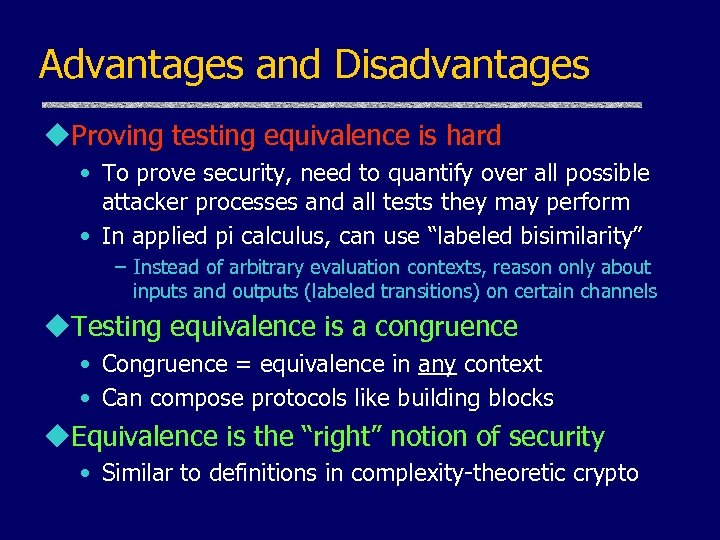Advantages and Disadvantages u. Proving testing equivalence is hard • To prove security, need to quantify over all possible attacker processes and all tests they may perform • In applied pi calculus, can use “labeled bisimilarity” – Instead of arbitrary evaluation contexts, reason only about inputs and outputs (labeled transitions) on certain channels u. Testing equivalence is a congruence • Congruence = equivalence in any context • Can compose protocols like building blocks u. Equivalence is the “right” notion of security • Similar to definitions in complexity-theoretic crypto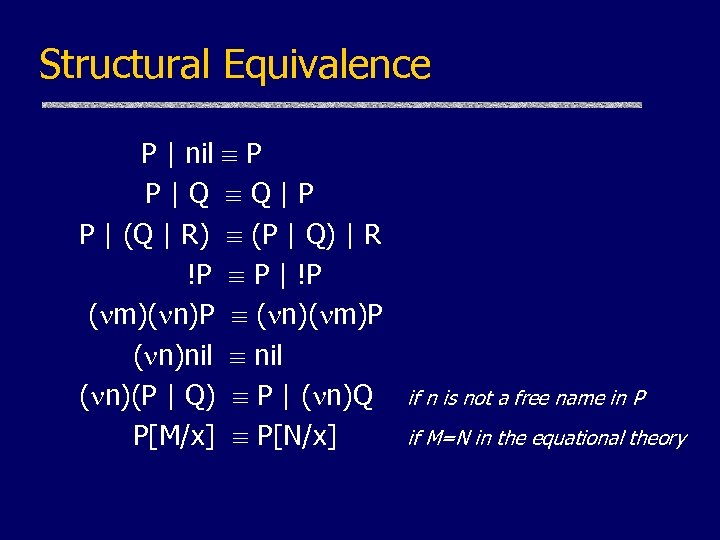Structural Equivalence P | nil P P|Q Q|P P | (Q | R) (P | Q) | R !P P | !P ( m)( n)P ( n)( m)P ( n)nil ( n)(P | Q) P | ( n)Q P[M/x] P[N/x] if n is not a free name in P if M=N in the equational theory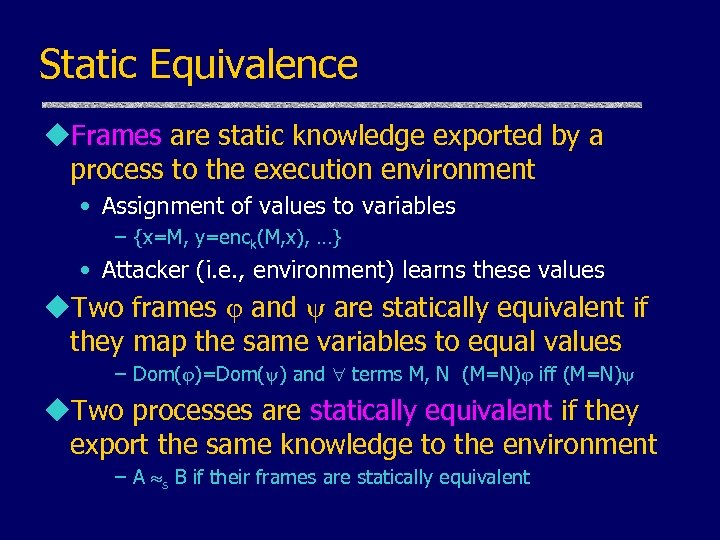Static Equivalence u. Frames are static knowledge exported by a process to the execution environment • Assignment of values to variables – {x=M, y=enck(M, x), …} • Attacker (i. e. , environment) learns these values u. Two frames and are statically equivalent if they map the same variables to equal values – Dom( )=Dom( ) and terms M, N (M=N) iff (M=N) u. Two processes are statically equivalent if they export the same knowledge to the environment – A s B if their frames are statically equivalent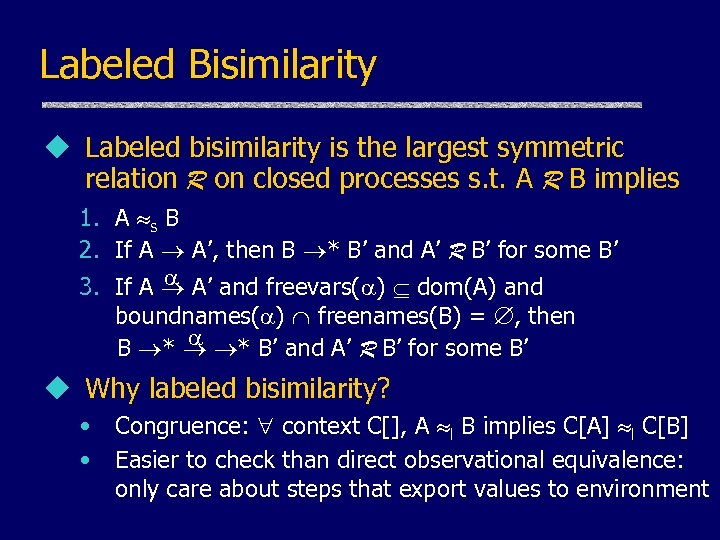Labeled Bisimilarity u Labeled bisimilarity is the largest symmetric relation R on closed processes s. t. A R B implies 1. A s B 2. If A A’, then B * B’ and A’ R B’ for some B’ 3. If A A’ and freevars( ) dom(A) and boundnames( ) freenames(B) = , then B * * B’ and A’ R B’ for some B’ u Why labeled bisimilarity? • • Congruence: context C[], A l B implies C[A] l C[B] Easier to check than direct observational equivalence: only care about steps that export values to environment